# Infrastructure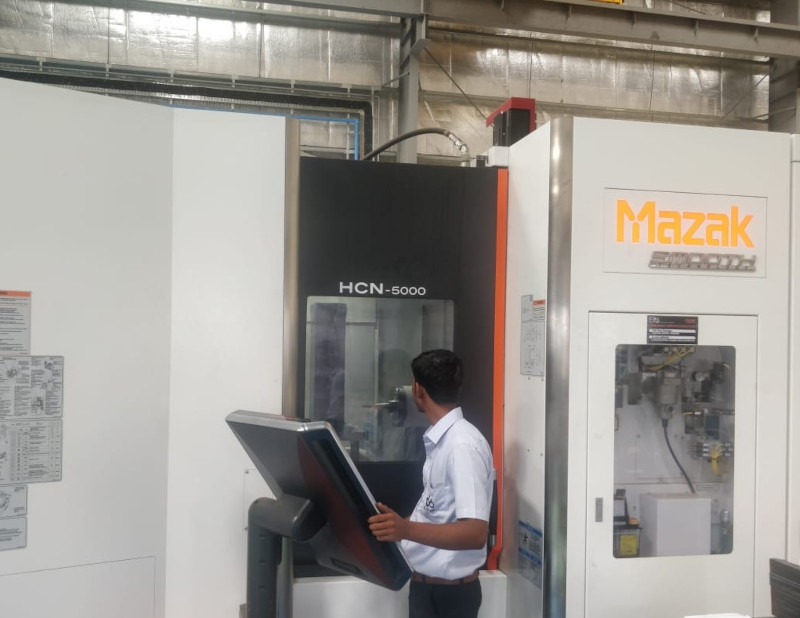HMC Machine
• Capacity: X=730mm, Y=830mm, Z=870mm
• Quantity: 1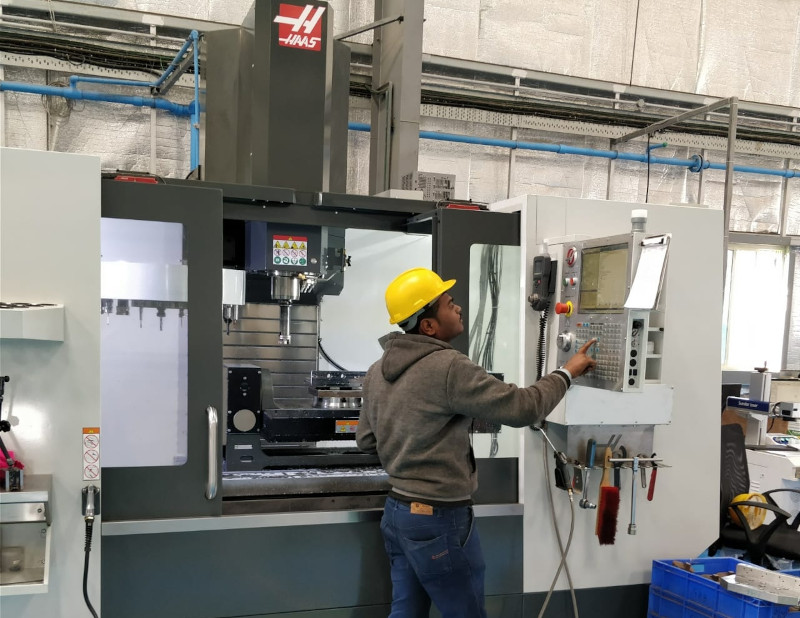5th AXIS VMC Machine
• Capacity: X=1270mm, Y=660mm, Z=635mm
•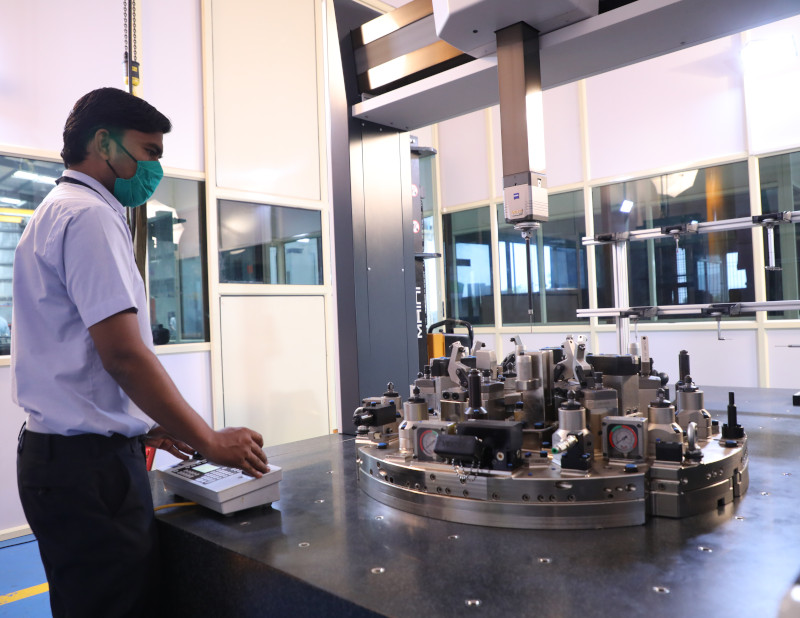Ziess Contura XT
• Capacity: X=1200mm, Y=2400mm, Z=1000mm
•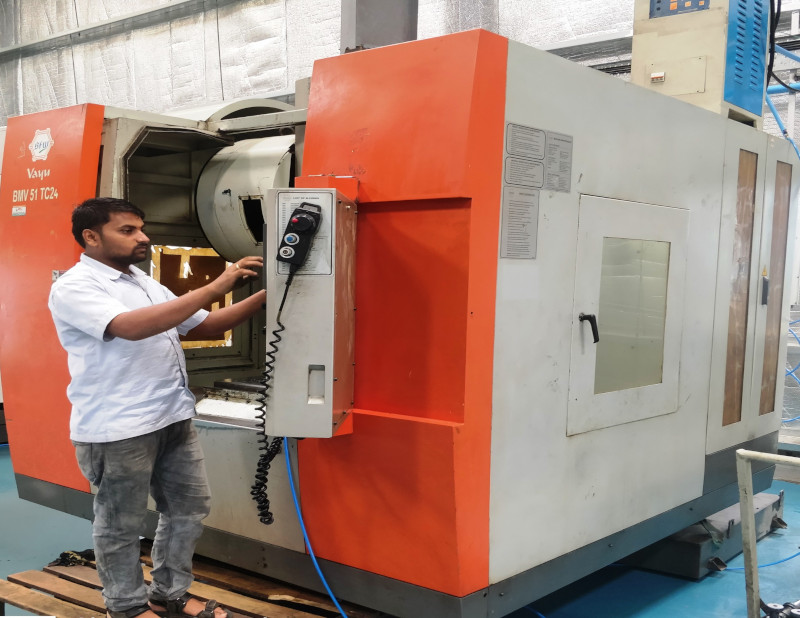BFW BMV 51 TC24 Vayu
• Machine Type: VMC
• Capacity: X=1250mm, Y=600mm, Z=600mm
• Quantity: 1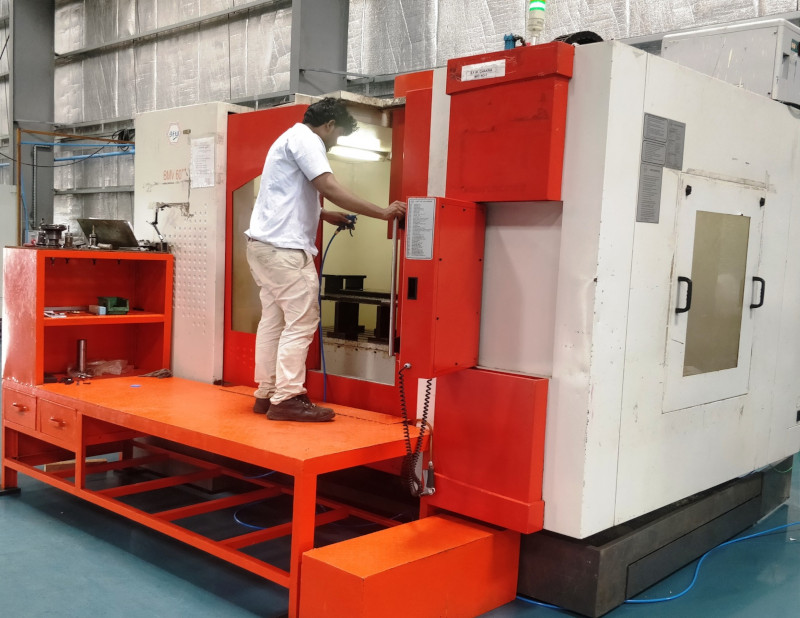BFW BMV 60++ Chakra
• Machine Type: VMC
• Capacity: X=1250mm, Y=600mm, Z=600mm
• Quantity: 1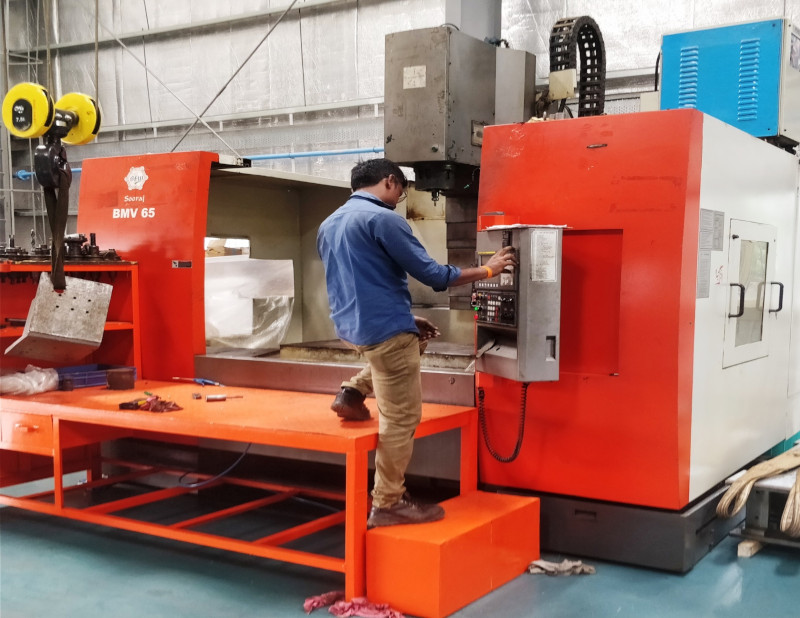BFW BMV 65 Sooraj
• Machine Type: VMC
• Capacity: X=1280mm, Y=700mm, Z=650mm
• Quantity: 1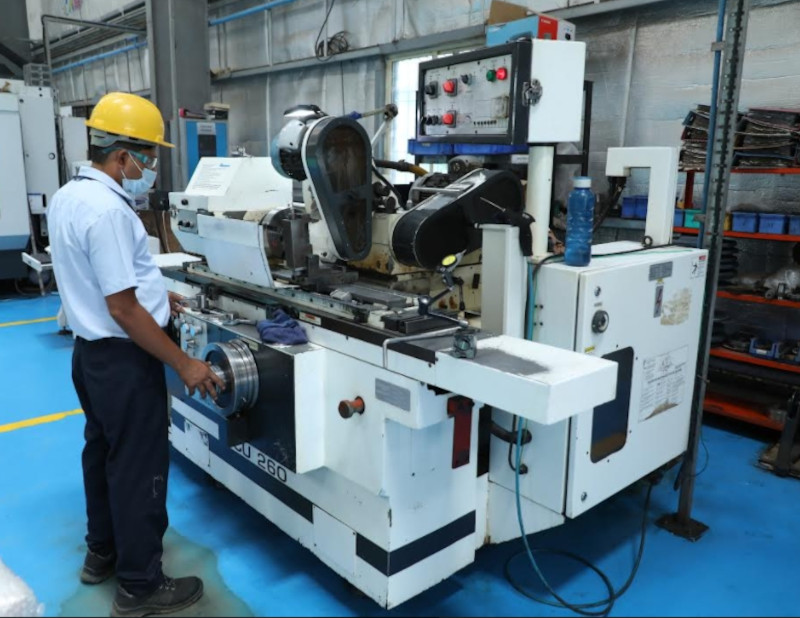Cylindrical Grinding - Proth PSGS 40100AHR (02 nos.)
•
•
•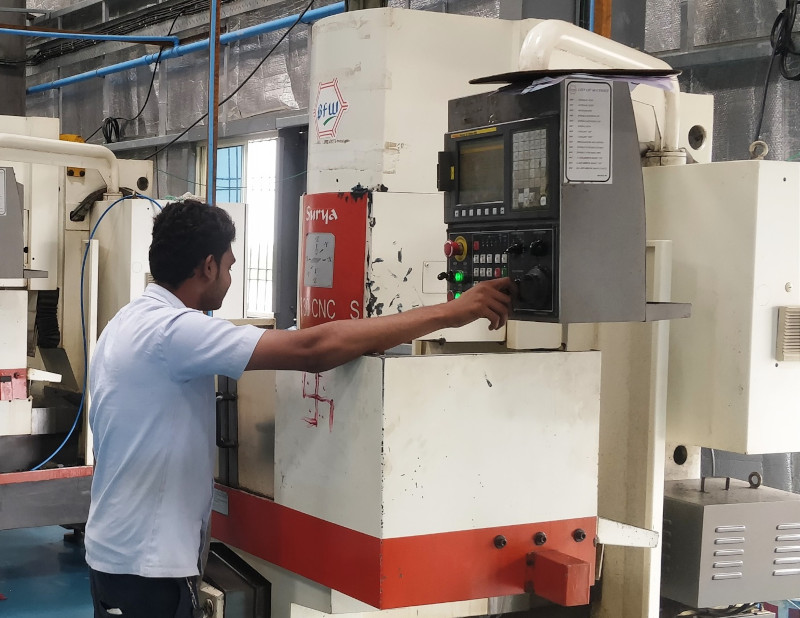BFW BMV VF30CNCVS Surya
• Machine Type: VMC
• Capacity: X=800mm, Y=350mm, Z=380mm
• Quantity: 4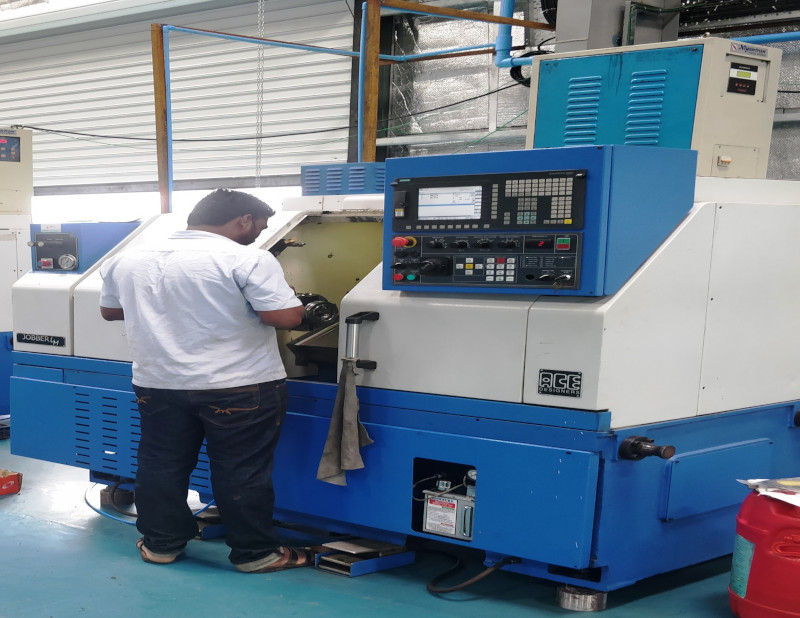Jobbler Junior
• Machine Type: CNC
• Capacity: X=345mm, Z=310mm
• Quantity: 1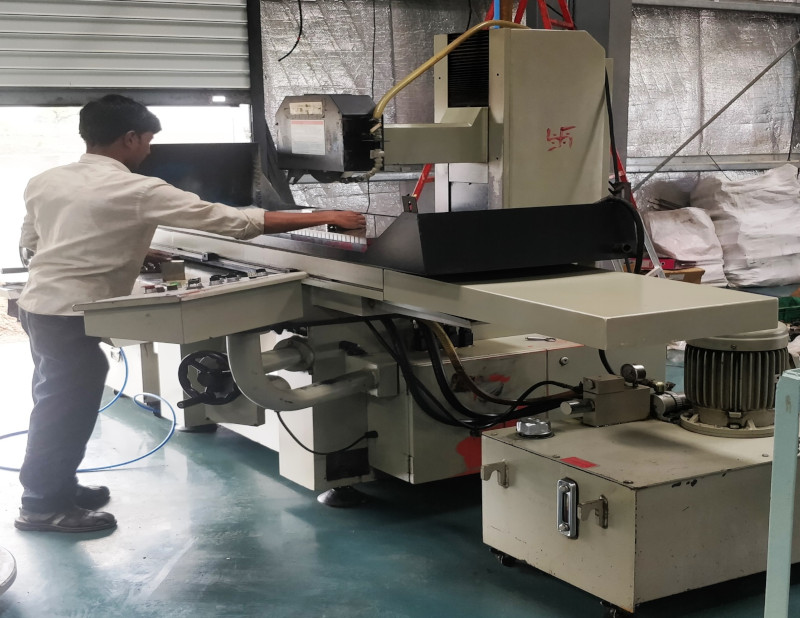Proth PSGS 40100AHR
• Machine Type: Surface Grinder
• Capacity: X=1000mm, Y=400mm, Z=600mm
• Quantity: 1